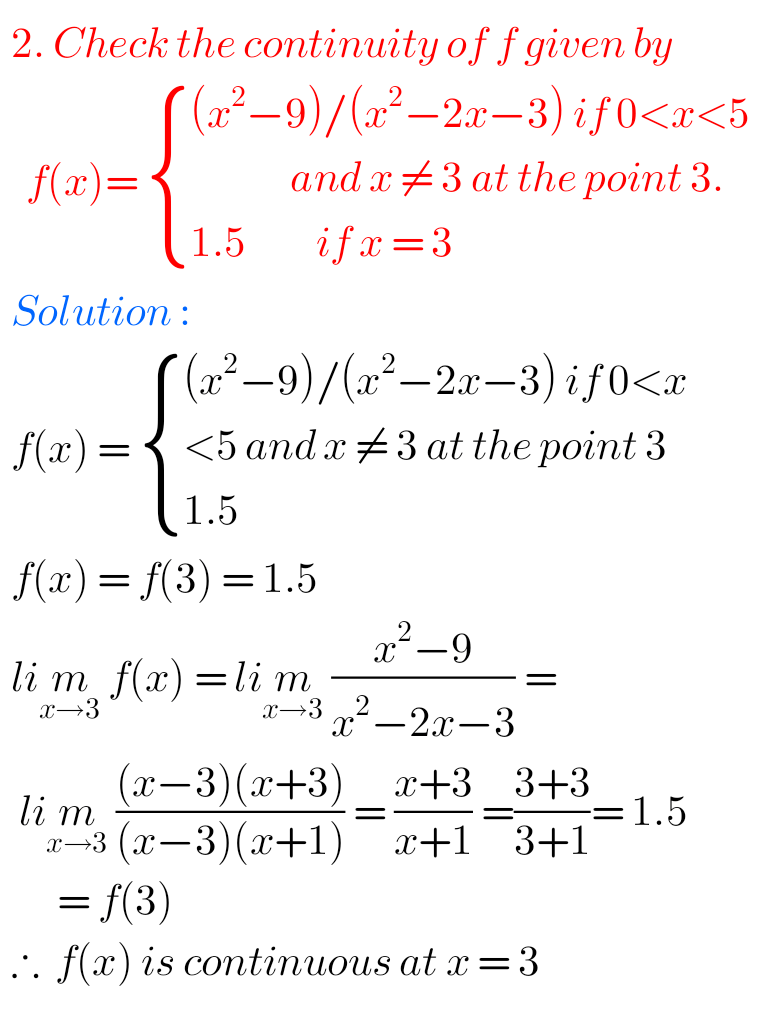## Inter Maths solutions for Limits and Continuity exercise 8(e)

Limits and Continuity Solutions Exercise 8(e) Inter Intermeduate Mathematics 1B textbook Chapter 8 Limits and Continuity Exercise 8(e)  solutions are given. First you sytudy the textbook lesson very well. Then observe the example problems and solutions given in the textbook. The keypoints in the lesson are very important. Observe the solutions given below and try …